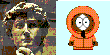David A. Kenny
August 2, 2021

Programs I Have Written

DyadR: twelve shiny apps for dyadic data analysis, one written with Rob Ackerman and another with Lara Stas, Axel Meyer, and Tom Loeys.

RDDD: three shiny apps for data restructuring of dyadic data written with Thomas Ledermann

SRM: Social Relations Model Programs

Other shiny Apps

MedPower (click to run): This app computes power for a simple three-variable (causal variable, variable, and outcome) mediation analysis using the combined power of paths a (the path from the causal variable to the mediator) and b (the path from the mediator to the outcome).

SVPower (click to run): This app conducts a power analysis taking account of the fact of heterogeneity, i.e., that effects vary from study to study.

QBASIC Programs

These are older programs that were written in QBASIC and are reposted.

relcomp (click to download): Reliability Computation -- Uses basic psychometric formulas to determine reliability. Does not input raw data. It computes

Cronbach's alpha from the number of items, the sum of the item variances and variance of the sum of the items.

Alpha from the average inter-item correlation and the number of items (i.e., the Spearman-Brown prophecy formula).

The number of items needed to achieve a given level of reliability given the average inter-item correlation.

Estimate the inter-item correlation from the alpha and the number of items.

A graph showing alpha a function of the number of items given the average inter-item correlation.

rtm (click to download): Regression Towards the Mean: Illustrates key ideas from the Campbell & Kenny book, A Primer on Regression Artifacts, including the Galton Squeeze diagram. The user is asked to roll 1 to 4 dice twice, creating a "pretest" and a "posttest." Shared dice create correlation. The program creates:

The means and standard deviations of the pretest and posttest and their correlation.

A scatterplot.

A Galton Squeeze diagram which more clearly illustrate regression towards the mean than a regression line.

Regression lines.

The means of the posttest for each score on the pretest and the means on the pretest for each score on the posttest.

Histograms for the pretest and posttest.